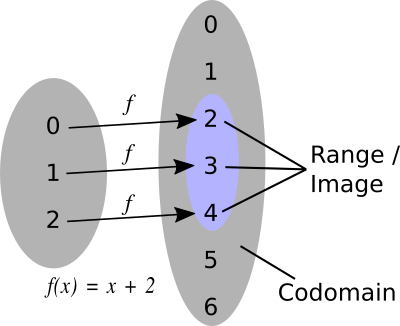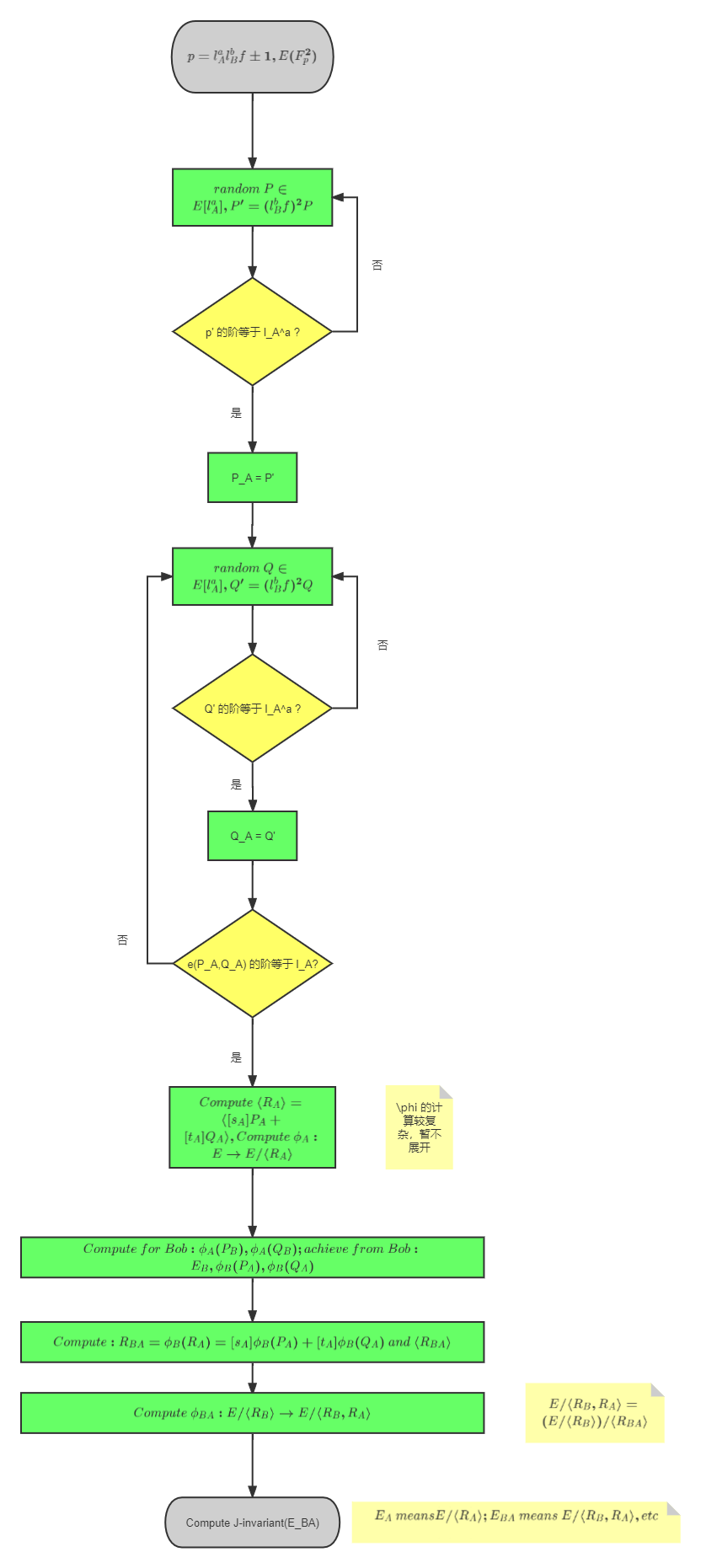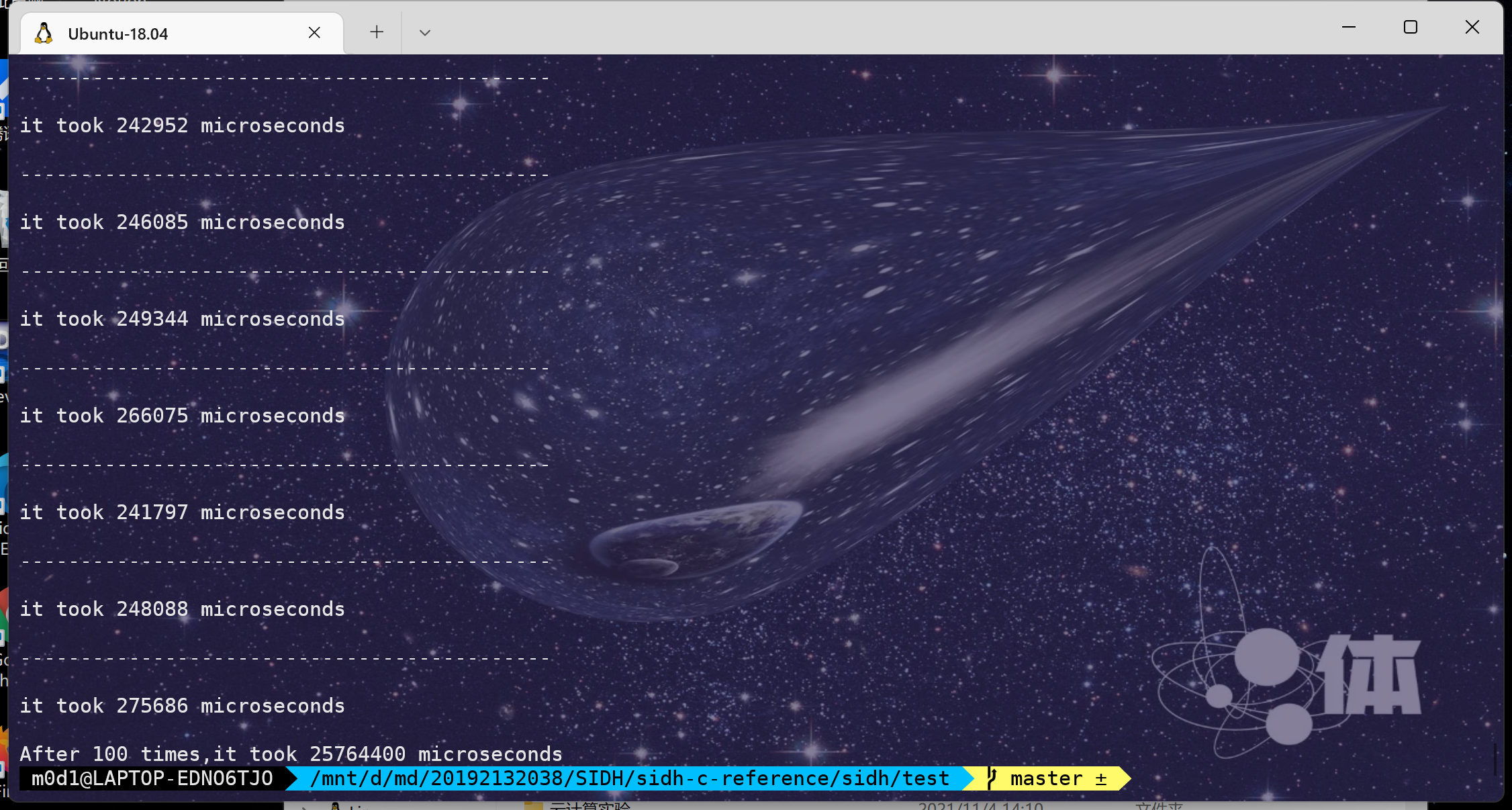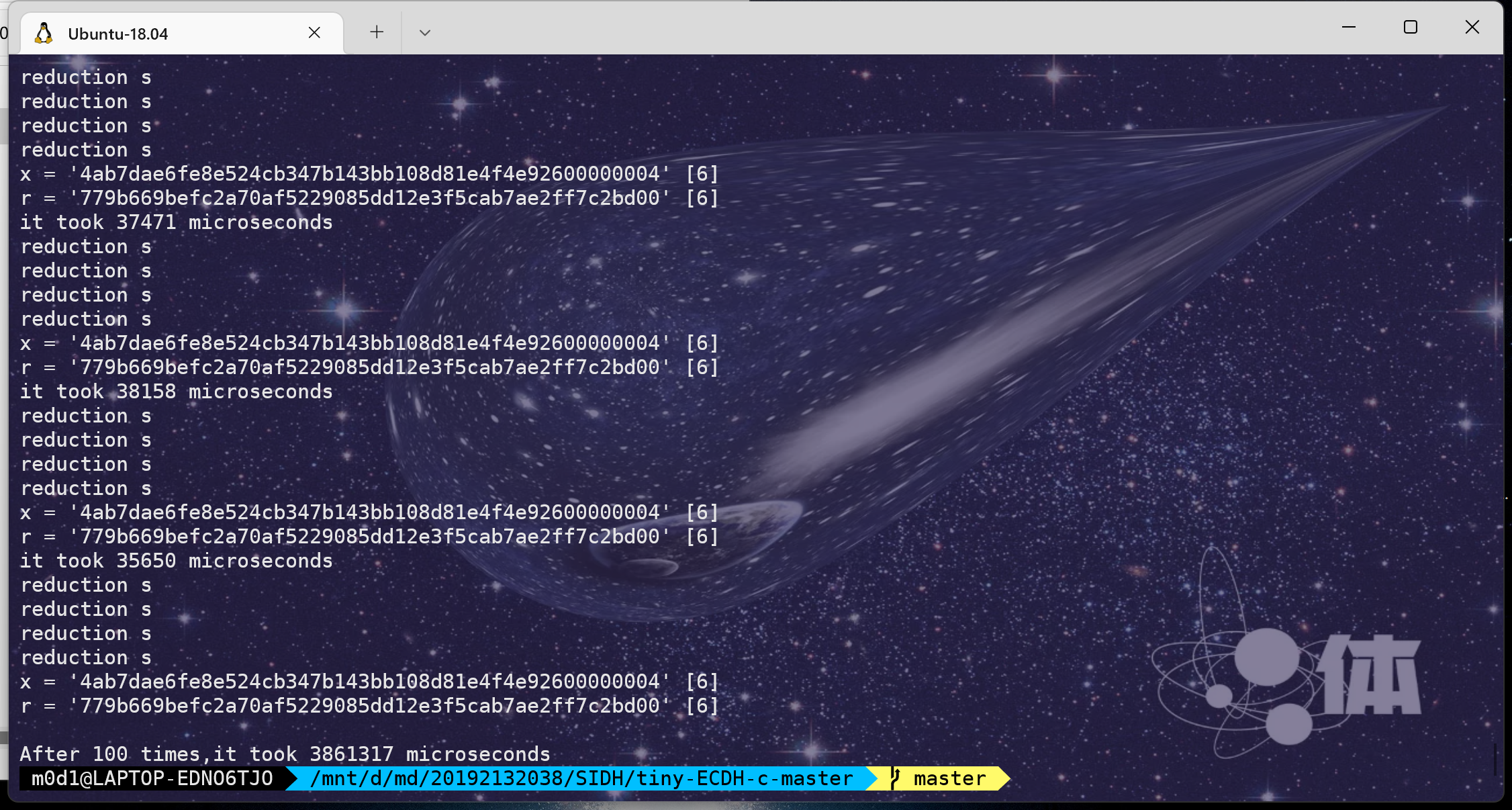【后量子密码】超奇异椭圆曲线同源密钥协商工程研究# 0.关键操作

0.复数运算（$F_{p^2}$ 里的任意元素长这样: $u+vi$ , 其中 $u,v \in F_p$ , $i^2 = -1$

1.素数判定（p是否为素数）

2.超奇异判定（E是否为超奇异椭圆曲线）

3.j-invariant计算

4.Weil pairing计算（为了检验P与Q是否线性无关）

5.倍点、点加、随机点的选取

6.复数运算

7.计算挠群

8.根据kernel计算同源映射(Velu’s formula)

9.等等……

SIDH最核心的一步就是计算同源（也是前量子公钥密码没有的操作）

## 0.1.Velu’s formula确定isogeny

### 0.1.0.准备工作

$\phi(x,y) = (\frac{x}{b},\frac{y}{b^2})$

$E_0:by^2 = x^3 + ax^2 + x$

$E_1:y^2+axy+by = x^3 + cx^2 + dx + e$

G,一个$E_1$的子群

### 0.1.1.分割G

1.丢弃单位元O

2.构造 $G_2$ 为 G，即G的2-挠群，R为剩下所有点的集合

3*.二等分R，分别命名为$R_+,R_-$ ，如果 $P \in R_+$ , 那么 $-P \in R_-$

### 0.1.2.计算系数

1.令 $S = R_+ \cup G_2,Q =(x_Q,y_Q) \in S$ ，计算：

• $f_Q = 3x_Q^2 + 2cx_Q + d - ay_Q$
• $g_Q = -2y_Q-ax_Q-b$
• 如果 $Q \in G_2$, 令 $v_Q = f_Q$，否则令 $v_Q = 2f_Q-ag_Q$
• $u_Q = (g_Q)^2$
• 计算 $v = \sum_{Q\in S}v_Q,w=\sum_{Q\in S}(u_Q+x_Qv_Q)$

### 0.1.3.构造codomain

$A = a$

$B = b$

$C = c$

$D = d-5v$

$E = e-(a^2+4c)v-7w$### 0.1.4.计算image

$\Phi: E_1 \rightarrow E_2$

$\Phi(x,y) = (\alpha,\beta)$

$\alpha = x+\sum_{Q\in S}(\frac{v_Q}{x-x_Q}-\frac{u_Q}{(x-x_Q)^2})$

$\beta = y - \sum_{Q\in S}(u_Q\frac{2y+ax+b}{(x-x_Q)^3}+v_Q\frac{a(x-x_Q)+y-y_Q}{(x-x-Q)^2}+\frac{au_Q-f_Qg_Q}{(x-x_Q)^2})$

# 1.程序流程图# 3.各种来源的代码分析

## 3.0.C/Cython/Python/Sagemath缝合代码

defeo/ss-isogeny-software: Software for “Quantum-Resistant Cryptosystems from Supersingular Elliptic Curve Isogenies” (github.com)

sagemath负责参数生成，python负责同源计算策略的计算，C负责关键算术运算（利用GMP库），密钥交换于Cython内完成

## 3.1.sidh-crypto的代码

sidh-crypto (github.com)

clear等释放内存的函数就不提及了

### curve.h:

#### 曲线相关

• 输入参数a和b，构造椭圆曲线：y^2 = x^3 + a * x^2 + b * x + c（这里选用的是Weierstrass form曲线，且域特征不等于2或3，且c=0）

• 求j-invariant

• 判断点是否落在曲线上

#### 点相关

• 生成零点、设置零点（即无穷远点）
• 指定生成一点（射影坐标，即有xyz三个参数）
• 令Q = P
• 判断点是否为零点（即无穷远点，z==0）
• 求逆元，即输入(x,y)输出(x,-y)
• 判断点的阶是否为2
• 判断两点是否相等
• 点加,有两种：输入有斜率；输入无斜率
• 点减，即input:P,Q ; output:P+(-Q)
• 倍点，有2倍点和任意倍
• 随机生成一点

### public_param.h

• 初始化公共参数（给它们赋值0）
• 从文件中读取公共参数
• 输出公共参数：$p,E,l_A,e_A,l_B,e_B$

### private_key.h

• 根据公钥生成私钥，大概就是由P和Q得到kernel的生成元R = [m]P+[n]Q,其中 $m\ mod\ l^e$ 存在逆元

### isogeny.h

• 用生成元计算isogeny
• 把domain的点P 同源映射 到codomain上，有两种方法：velu和kohel
• 计算kernel的partition
• 计算共享曲线E，有两种：naive和strategy，naive的最后大概要650000ms，strategy的话大概是250000ms

## 3.2.Microsoft的代码

microsoft/PQCrypto-SIDH

# 4.运行时间测量与比较kokke/tiny-ECDH-c# 5.优化方向

（1）有限域的运算：任何加速底层的有限域的基本算术方法都能加快SIDH的实现。

（2）标量乘：即倍点运算

（3）曲线以及坐标的类型：不同的曲线模型以及不同的坐标形式，其上的点加、倍点、同源和同源曲线的代数操作的计算量是不同的

（4）同源和同源曲线的计算：在SIDH中，Alice和Bob均需要计算$l^e$-同源和同源曲线。对于$l^e$-同源的计算，需要进行e次 $l$-同源的复合。显然复合次数越少，计算速度越快。对于同源曲线的计算，当 $l$ 较大时，直接利用同源曲线公式计算效率较低。

（5）压缩公钥和恢复公钥的计算量：暂时忽略。

# 参考

 Efficient Implementations of A Quantum-Resistant Key-Exchange Protocol on Embedder systems

 Efficient algorithms for supersingular isogeny Diffie-Hellman

 A Friendly Introduction to Supersingular Isogeny Diffie-Hellman

 椭圆曲线同源的有效计算研究进展

 Quantum Computation and Quantum Information